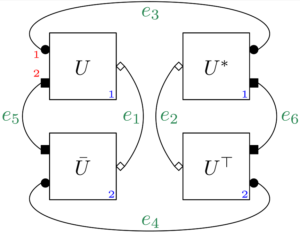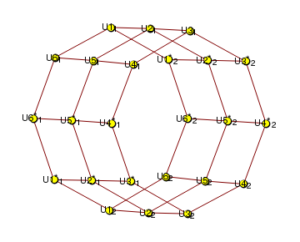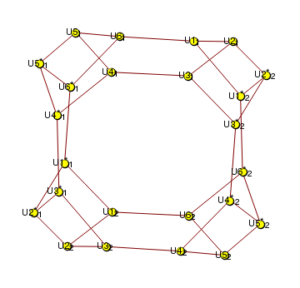# RTNI – A symbolic integrator for Haar-random tensor networks

Together with Motohisa Fukuda and Robert König we have a new paper (and some code!) RTNI – A symbolic integrator for Haar-random tensor networks. This is a very unusual paper for me: the main goal here is not to prove theorems but to introduce a piece of software we developed: RNTI. RTNI is a software package, available for Mathematica and for python, which computes the expectation value of random tensors defined by random, Haar-distributed, unitary operators.

{Update [07/06/2019]: Moto Fukuda has developed a web-based light version of RTNI, see here}

Let us unpack all these technical terms. First, unitary operators: these are linear transformations (or matrices) which are rotation-like, meaning that they are invertible and they do not modify the magnitude of the vectors they act on. Here is a counterclockwise 45° (or $\pi/4$) rotation: $U_1 = \frac{1}{\sqrt 2}\begin{bmatrix} 1 & 1 \\ 1 & -1 \end{bmatrix}$ and below is a unitary matrix realizing the symmetry with respect to the first coordinate axis in a plane
$U_2 = \begin{bmatrix} 1 & 0 \\ 0 & -1 \end{bmatrix}.$

In the general case, $d \times d$ unitary matrices satisfy the condition $UU^*=U^*U=I_d$, where the star operation corresponds to taking the (Hermitian) adjoint (complex conjugation, followed by transposition). The set of unitary matrices form a compact group under multiplication, and this group admits a unique probability measure which is invariant under left/right translations by group elements: the Haar measure. As it turns out, in the case of the unitary group (and even more so for its relatives, the orthogonal and the symplectic groups), the Haar measure is complicated, and computing integrals with respect to it is a daunting task.

But before we get into computing integrals with respect to the Haar measure, let me address an even more fundamental question regarding it: sampling. Producing a Haar-distributed random unitary matrix on a computer is quite easy: construct a random matrix with enough symmetry (say, a Ginibre or a GUE matrix), compute its singular value/eigenvalue decomposition, and just take one of the unitary matrices appearing in that decomposition. The initial symmetry of your random matrix will translate into the invariance property that uniquely identifies the Haar measure (although there are some minor subtleties, see here or here). Numerous software implementations of this procedure exist, here is one example in a MATLAB library popular with the Quantum Information Theory crowd.

Once that we have properly defined the Haar measure and that we know how to produce samples from it, the next question that comes to mind is how to compute integrals with respect to it. This problem was first considered in the physics literature by Weingarten in the asymptotic limit of large dimension. The mathematical theory was developed, rigorously and in full generality, by Benoit Collins (here, and later with Piotr Śniady), who also coined the name Weingarten formula for the following result.

Theorem [Weingarten formula] Let $d,p$ be positive integers and $i=(i_1,\ldots ,i_p)$, $i’=(i’_1,\ldots ,i’_p)$, $j=(j_1,\ldots ,j_p)$, $j’=(j’_1,\ldots ,j’_p)$ be $p$-tuples of positive integers from $\{1, 2, \ldots, d\}$. Then
$\int_{\mathcal U(d)}U_{i_1j_1} \cdots U_{i_pj_p} \bar U_{i’_1j’_1} \cdots \bar U_{i’_pj’_p} \mathrm{d}U=\!\!\! \sum_{\alpha, \beta\in \mathcal S_{p}}\delta_{i_1i’_{\alpha (1)}}\ldots \delta_{i_p i’_{\alpha (p)}}\delta_{j_1j’_{\beta (1)}}\ldots \delta_{j_p j’_{\beta (p)}} \mathrm{Wg}_d (\alpha^{-1}\beta).$ If $p\neq p’$ then
$\int_{\mathcal U(d)}U_{i_{1}j_{1}} \cdots U_{i_{p}j_{p}} \bar U_{i’_{1}j’_{1}} \cdots \bar U_{i’_{p’}j’_{p’}}\mathrm{d}U= 0.$ Here we denoted by $\mathcal U(d)$ the unitary group acting on an $d$-dimensional Hilbert space, and by $\mathcal S_p$ the symmetric group on $p$ elements. The integrals are taken with respect to the normalized Haar measure on $\mathcal U(d)$. The function $\mathrm{Wg}_d$ is called the unitary Weingarten function.

The above formula allows one to compute the expectation of any monomial (and thus, by linearity, any polynomial) in the entries of a Haar-distributed random unitary matrix. But there is one caveat: the Weingarten function appearing in the formula above is an object of combinatorial nature, which has no explicit formula for general $d$. Nonetheless, for fixed monomial order $p$, there exist algorithmic procedures to compute the values $\mathrm{Wg}_d(\sigma)$ for all permutations $\sigma \in \mathcal S_p$ and thus one can compute averages of monomials such as
$\mathbb{E} |U_{11}|^2 |U_{22}|^2 = \mathrm{Wg}_d((1)(2)) = \frac{1}{d^2-1} \qquad \quad \mathbb{E} U_{11} U_{22} \bar U_{12} \bar U_{21} = \mathrm{Wg}_d((12)) = \frac{-1}{d(d^2-1)}.$

As it turns out, in practical applications, one does not encounter often polynomials in the entries of a random unitary matrix $U$. It is more likely to be faced with expressions which involve (traces of) polynomials in the matrix $U$ itself, together with some other given matrices. For instance, the following type of expression is quite common (in, e.g., Quantum Information Theory): $\mathbb{E} \operatorname{Tr}[XUYU^*]$, where $X,Y$ are two given, fixed $d \times d$ matrices. One could, of course, write the trace in coordinates, and compute the expectation as a sum where the individual terms are calculated with the help of the Weingarten formula. Here’s where RTNI comes to the rescue, saving you all that trouble (we use here the Mathematica implementation of RTNI):

In:= MultinomialexpectationvalueHaar[d, {1, 2}, {X, Y}, True] Out= (Tr[X] Tr[Y])/d

Above, the MultinomialexpectationvalueHaar function computes $\mathbb E \operatorname{Tr}[X U^{(i_1)} Y U^{(i_2)}]$, where $i_1 = 1$ (meaning we simply take $U$) and $i_2 = 2$ (meaning we take $U^*$). Using this function, one can compute (the trace of) any linear expression involving Haar-distributed random unitary matrices $U,\bar U, U^*, U^\top$ and deterministic matrices.

But RTNI can do much more than that! It can compute expectation values of (non-linear) graphs of matrices (i.e. tensors) involving random unitary matrices which can act on tensor products of vector spaces. Such situations are very common in quantum information theory and in theoretical physics in general, where networks of tensors are becoming very popular. One starts from the description $G$ of a tensor in Penrose graphical notation; $G$ is a graph where the vertices correspond to tensors, and edges correspond to tensor contraction. Importantly, the tensor product operation corresponds to the disjoint union of graphs. Here is an example coming from the theory of random quantum channels. Consider a random quantum channel given by a Haar-distributed random isometry $V: \mathbb C^d \to \mathbb C^k \otimes \mathbb C^n$ ($V$ is just the truncation to the first $d \leq kn$ columns of a $kn \times kn$ random unitary matrix $U$)
$\Phi: M_d(\mathbb C) \to M_k(\mathbb C), \qquad \Phi(X) = [\mathrm{id}_k \otimes \operatorname{Tr}_n](VXV^*).$ Such random quantum channels were first considered by Hayden and Winter in relation to the additivity conjecture in quantum information theory, solved in the negative by Hastings. In such considerations, one bounds the minimum output entropy of the tensor product channel $\Phi \otimes \bar \Phi$ by using the overlap
$f:=\operatorname{Tr}[\omega_k \cdot [\Phi \otimes \bar \Phi](\omega_d)]$ between the output of the channels acting on a maximally entangled state $\omega_d$ with another maximally entangled state (on the output spaces) $\omega_k$. Recall that the maximally entangled state is defined, in general, by $\omega_n := \Omega_n \Omega_n^*$, where the vector $\Omega_n \in \mathbb C^d \otimes \mathbb C^d$ reads $\Omega_n:= \frac{1}{\sqrt n} \sum_{i=1}^n e_i \otimes e_i,$ with $\{e_1, \ldots, e_n\}$ being an orthonormal basis of $\mathbb C^n$. Here $\bar \Phi$ is the complex conjugate channel, obtained by taking the entry-wise conjugate of $\Phi$. The diagram for the scalar $f$ is given belowOne can input the graph above into RTNI using the following Mathematica commands:

In:= e1 = {{"U*", 2, "out", 1}, {"U", 1, "in", 1}}; In:= e2 = {{"U*", 1, "out", 1}, {"U", 2, "in", 1}}; In:= e3 = {{"U", 1, "out", 1}, {"U*", 1, "in", 1}}; In:= e4 = {{"U", 2, "out", 1}, {"U*", 2, "in", 1}}; In:= e5 = {{"U", 1, "out", 2}, {"U*", 2, "in", 2}}; In:= e6 = {{"U", 2, "out", 2}, {"U*", 1, "in", 2}}; In:= g = {e1, e2, e3, e4, e5, e6}; In:= listg = {{g, 1/(d k)}};

Note that the final command assigns a weight $1/(dk)$ to the graph $g$; this corresponds to the normalization of the two maximally entangled states $\omega_d, \omega_k$. The edge numbering in the code matches the numbering in the picture, as do the numberings of the different $U$ boxed and of the tensor factors. One has to call then the integrateHaarUnitary function of the RTNI package to compute the expected value of the scalar $f$ defined above with respect to the Haar measure on $\mathcal U(kn)$. The routine integrateHaarUnitary implements the graphical Weingarten calculus which I developed together with Benoit in this paper. It is basically the Weingarten formula, read in the Penrose graphical calculus. RTNI gives the following output, which we further manipulate in order to obtain the asymptotic overlap in the regime where $d \sim tkn$:

In:= Eg = integrateHaarUnitary[listg, "U", {d}, {n, k}, n k] In:= overlap = Eg[[1, 2]]; In:= overlapt = overlap /. {d -> t n k}; In:= Assuming[t > 0 && k > 1, Limit[overlapt, n -> Infinity]] // Simplify Out:= {{{}, -((d^2 k^2 n)/(d k - d k^3 n^2)) - (d k n^2)/( d k - d k^3 n^2) + (d k^2 n)/(d k^2 n - d k^4 n^3) + (d^2 k n^2)/( d k^2 n - d k^4 n^3)}} Out:= (1 - t)/k^2 + t

This study case emphasizes the strengths of the RTNI package: computing the expected overlap $f$ using the algebraic Weingarten formula would have required tedious summations and case analysis for index collisions. The RTNI package provides a simple, conceptual way of computing such expectations. RTNI can also display the different tensor networks one inputs, here are some examples:If you are interested in using RTNI for your research, we would be happy to hear about it. Also, if you want to implement the killer feature of RTNI which is missing in the current release, please contact us!